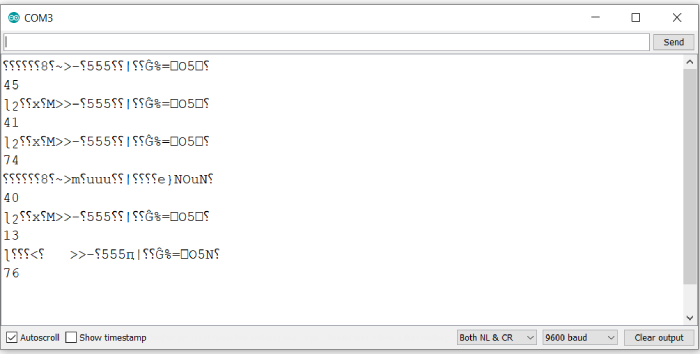# Generate random numbers in Arduino

Generating random numbers is one of the key requirements from microcontrollers. Random numbers have several applications. Let’s not get there. You must have an application in mind, which brought you to this page. Generating random numbers is very easy in Arduino, thanks to the inbuilt random() function.

## Syntax

random(min, max)

OR

random(max)

where min is 0 by default.

Min is inclusive, while max is exclusive. Thus, random(10,50) will return a number integer between 10 and 49 (10 and 49 included). random(100) will return a random number between 0 and 99, both included. Note that the random function’s return type is long.

## Example

void setup() {
// put your setup code here, to run once:
Serial.begin(9600);
Serial.println();
long r1 = random(100);
Serial.println(r1);
}

void loop() {
// put your main code here, to run repeatedly:
}

## Output

The Serial Monitor output is shown below −Ignore the garbage output. Every time the board is reset, some garbage gets printed. But you can see that the random number printed every time is different.